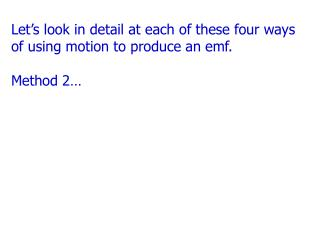DownloadDownload PresentationLet’s look in detail at each of these four ways of using motion to produce an emf. Method 2…

# Let’s look in detail at each of these four ways of using motion to produce an emf. Method 2…

Télécharger la présentation## Let’s look in detail at each of these four ways of using motion to produce an emf. Method 2…

- - - - - - - - - - - - - - - - - - - - - - - - - - - E N D - - - - - - - - - - - - - - - - - - - - - - - - - - -
##### Presentation Transcript

1. Let’s look in detail at each of these four ways of using motion to produce an emf. Method 2…

2. B B                                                             Another Kind of Generator: A Slidewire Generator Recall that one of the ways to induce an emf is to change the area of the loop in the magnetic field. Let’s see how this works. v A U-shaped conductor and a moveable conducting rod are placed in a magnetic field, as shown. ℓ The rod moves to the right with a constant speed v for a time dt. dA vdt x The area through which flux passes increases by dA.

3. The loop is perpendicular to the magnetic field, so the magnetic flux through the loop is B = = BA. Calculate the induced emf using Faraday’s law: v B B                         ℓ                         dA             vdt x B and v are vector magnitudes, so they are always +. Wire length is always +. You use Lenz’s law to get the direction of the current.

4. B                               Direction of current? The induced emf causes current to flow in the loop. Magnetic flux inside the loop increases (more area). v System “wants” to make the flux stay the same, so the current gives rise to a field inside the loop into the plane of the paper (to counteract the “extra” flux). ℓ I dA vdt x Clockwise current! What would happen if the bar were moved to the left?

5. As the bar moves through the magnetic field, it “feels” a force v B       A constant pulling force, equal in magnitude and opposite in direction, must be applied to keep the bar moving with a constant velocity. FM FP             ℓ             I x

6. Power and current. If the loop has resistance R, the current is v B       And the power is             ℓ             I (as expected). x Mechanical energy (from the pulling force) has been converted into electrical energy, and the electrical energy is then dissipated by the resistance of the wire.

7. You might find it useful to look at Dr. Waddill’s lecture on Faraday’s Law, from several semesters back. Click here to view the lecture. If the above link doesn’t work, try copying and pasting this into your browser address bar: http://campus.mst.edu/physics/courses/24/Handouts/Lec_18.ppt